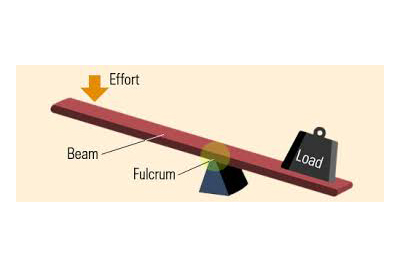Levers - Physics
09-11-2018    1156 times### Levers

Levers are the simple machines which used in our daily life. Most of the case, we don’t know about these machines but we used it.

#### What is a Lever?

A rigid, straight (or bent) bar, which is capable of turning about a fixed axis (fulcrum), is known as lever.

#### What is a fulcrum?

The axis, about which the lever turns, passes through a point of the lever which is known as fulcrum. In generally it marked by the letter F. when the lever in used then this fulcrum remains fixed.

Let a straight rod AB with the fulcrum point F. Another two points place within the straight line AB, Force or effort (E) and Load (L).  The distance between the fulcrum (F) and Load (L) is known as load arm. And the distance between the fulcrum (F) and Force (E) effort arm.

It is assumed that the lever is weightless and frictionless. That type of levers known as ideal lever. It works on the principal of momentum. In the equilibrium position of the lever, by the principal of moments,